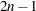The VARCLUS Procedure
 Output Data Sets

### OUTSTAT= Data Set

The OUTSTAT= data set is TYPE=CORR, and it can be used as input to the SCORE procedure or a subsequent run of PROC VARCLUS. The OUSTAT= data set contains the following variables:

• BY variables

• _NCL_, a numeric variable giving the number of clusters

• _TYPE_, a character variable indicating the type of statistic the observation contains

• _NAME_, a character variable containing a variable name or a cluster name, which is of the form CLUS, whereis the number of the cluster

• the variables that are clustered

The values of the _TYPE_ variable are listed in the following table.

Table 94.2 _TYPE_

_TYPE_

Contents

MEAN

means

STD

standard deviations

USTD

uncorrected standard deviations, produced when the NOINT option is specified

N

number of observations

CORR

correlations

UCORR

uncorrected correlation matrix, produced when the NOINT option is specified

MEMBERS

number of members in each cluster

VAREXP

variance explained by each cluster

PROPOR

proportion of variance explained by each cluster

GROUP

number of the cluster to which each variable belongs

RSQUARED

squared multiple correlation of each variable with its cluster component

SCORE

standardized scoring coefficients

USCORE

scoring coefficients to be applied without subtracting the mean from the raw variables, produced when the NOINT option is specified

STRUCTUR

cluster structure

CCORR

correlations between cluster components

The observations with _TYPE_="MEAN", "STD", "N", and "CORR" have missing values for the _NCL_ variable. All other values of the _TYPE_ variable are repeated for each cluster solution, with different solutions distinguished by the value of the _NCL_ variable. If you want to specify the OUTSTAT= data set with the SCORE procedure, you can use a DATA step to select observations with the _NCL_ variable missing or equal to the desired number of clusters as follows:

```data Coef2;
set Coef;
if _ncl_ = . or _ncl_ = 3;
drop _ncl_;
run;

proc score data=NewScore score=Coef2; run;
```

PROC SCORE standardizes the new data by subtracting the original variable means that are stored in the _TYPE_=’MEAN’ observations, and dividing by the original variable standard deviations from the _TYPE_=’STD’ observations. Then PROC SCORE multiplies the standardized variables by the coefficients from the _TYPE_=’SCORE’ observations to get the cluster scores.

### OUTTREE= Data Set

The OUTTREE= data set contains one observation for each variable clustered plus one observation for each cluster of two or more variables—that is, one observation for each node of the cluster tree. The total number of output observations is betweenand, whereis the number of variables clustered.

The OUTTREE= data set contains the following variables:

• BY variables, if any

• _NAME_, a character variable giving the name of the node. If the node is a cluster, the name is CLUS, whereis the number of the cluster. If the node is a single variable, the variable name is used.

• _PARENT_, a character variable giving the value of _NAME_ of the parent of the node. If the node is the root of the tree, _PARENT_ is blank.

• _LABEL_, a character variable giving the label of the node. If the node is a cluster, the label is CLUS, whereis the number of the cluster. If the node is a single variable, the variable label is used.

• _NCL_, the number of clusters

• _VAREXP_, the total variance explained by the clusters at the current level of the tree

• _PROPOR_, the total proportion of variance explained by the clusters at the current level of the tree

• _MINPRO_, the minimum proportion of variance explained by a cluster component

• _MAXEIG_, the maximum second eigenvalue of a clusterPrevious Page | Next Page | Top of Page